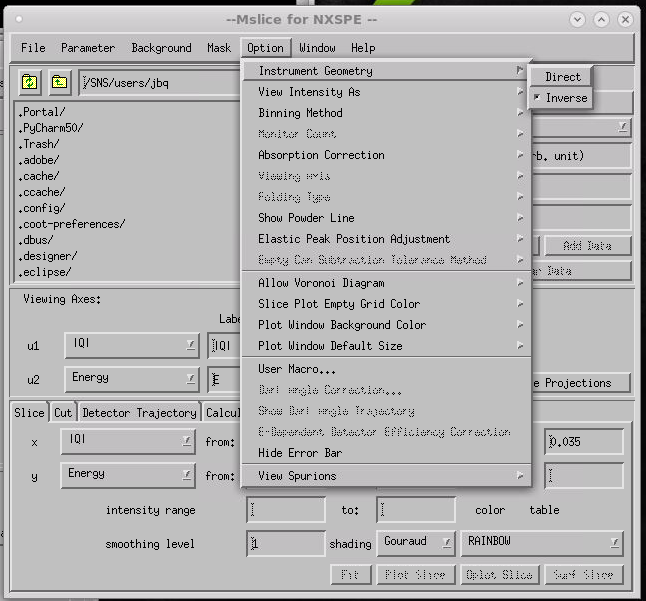$$\renewcommand\AA{\unicode{x212B}}$$

# BASISReduction v1¶

## Summary¶

Multiple-file BASIS reduction for its two reflections.

## Properties¶

Name

Direction

Type

Default

Description

RunNumbers

Input

string

Sample run numbers

DoIndividual

Input

boolean

False

Do each run individually

ExcludeTimeSegment

Input

string

Exclude a contigous time segment; Examples: “71546:0-60” filter run 71546 from start to 60 seconds, “71546:300-600”, “71546:120-end” from 120s to the end of the run

RetainTimeSegment

Input

string

Only retain events occurring within a time segment. Examples: 71546:0-3600 only retains events from the first hour of run 71546. 71546:3600-7200 retain only the second hour. 71546:7200-end retain events after the first two hours

GroupDetectors

Input

string

None

Switch for grouping detectors. Allowed values: [‘None’, ‘Low-Resolution’, ‘By-Tube’]

DoFluxNormalization

Input

boolean

True

Do we normalize data by incoming flux?

FluxNormalizationType

Input

string

Monitor

Flux Normalization Type. Allowed values: [‘Monitor’, ‘Proton Charge’, ‘Duration’]

NormalizeToFirst

Input

boolean

False

Normalize spectra to intensity of spectrum with lowest Q?

ReflectionType

Input

string

silicon_111

Analyzer. Documentation lists typical associated property values. Allowed values: [‘silicon_111’, ‘silicon_311’, ‘silicon_333’]

EnergyBins

Input

dbl list

-150,0.4,500

Energy transfer binning scheme (in ueV)

MomentumTransferBins

Input

dbl list

0.3,0.2,1.9

Momentum transfer binning scheme

Input

string

See documentation for latest mask files. Allowed extensions: [‘.xml’]

Input

boolean

False

Do we normalize by the vanadium intensity?

NormalizationType

Input

string

by Q slice

Select a Vanadium normalization. Allowed values: [‘by Q slice’, ‘by detector ID’]

NormRunNumbers

Input

string

Normalization run numbers

SaveNXSPE

Input

boolean

False

Do we save to NXSPE format?

PsiAngleLog

Input

string

SE50Rot

name of entry in the logs storing the psi angle

PsiOffset

Input

number

0

add this quantity to the psi angle stored in the log

OutputSusceptibility

Input

boolean

False

Output dynamic susceptibility (Xqw)

OutputPowderSpectrum

Input

boolean

False

Output S(Q) and S(theta) powder diffraction

RemoveTemporaryWorkspaces

Input

boolean

True

Remove temporary workspaces and files

For each property, the algorithm will remember the last value used. If user deletes this value and leaves blank the property field, the default value will be used. Default values are typical of the silicon111 reflection.

## Description¶

Run numbers: Reduction can be carried out for each run or all runs can be aggregated into a single data collection.

Examples:

• 2144-2147: runs from 2144 to 2147.

• 2144-2147,2149,2156: runs from 2144 to 2147 and also runs 2149 and 156

If DoIndividual is checked, then each run number is reduced separately from the rest.

ExcludeTimeSegment: Events happening in a time segment with no proton charge are most likely noise. Those events can be filtered out of the reduction process.

Example:

• “71465:0-500,71466:900-2100,71467:4000-end” will filter out events happening between the start of the run and 500 seconds for run 71465, then between 900 and 2100 seconds for run 71466 and between 4000 seconds and the end of the run for 71467. Only one time segment can be excluded per run number.

RetainTimeSegment: When interested only in a single and contiguous part of the run. Only events within that time window can be kept.

Examples: - “71465:0-3600,71466:3600-7200,71467:3600-end” will retain events of the first hour of run 71465, events of the second hour of run 71466, and events after the first hour of run 71467. Events outside these time windows will not be taken into account.

Momentum transfer binning scheme: Three values are required, the center of the bin with the minimum momentum, the bin width, and the center of the bin with the maximum momentum.

Rescaling to first spectrum: Since the Y-scale has arbitrary units, a rescaling convention is taken whereby the maximum of the first spectrum (lowest Q-value) is rescaled to 1.0. This rescaling may not be employed when the intent is to compare to other runs, like can substraction of comparison between deuterated and hydrogenated samples.

### Reflection Selector¶

Currently three types of reflection are possible, associated with the silicon analyzers of BASIS. These are typical binning values for each reflection:

Reflection

Energy bins

(micro-eV)

Momentum transfer bins

(inverse Angstroms)

silicon111

-150, 0.4, 500

0.3, 0.2, 1.9

silicon311

-740, 1.6, 740

0.5, 0.2, 3.7

silicon333

-1500, 3.2 1500

0.5, 0.2, 3.7

Also the following default mask files are associated to each reflection:

Reflection

silicon111

silicon311

silicon333

Note: masks for reflections 111 and 333 are actually the same since both reflections take place at the same silicon crystal analyzers.

The syntax for the vanadium run numbers designation (NormRunNumbers) is the same as in the case of the sample (hyphens and commas are understood). All runs are jointly reduced into a single vanadium workspace.

Normalization type by Q slice is the default normalization. In this case, the sample is reduced into $$S_{s}(Q,E)$$ and the vanadium is reduced into $$S_{v}(Q,E)$$. Later, $$S_{v}(Q,E)$$ is integrated along the energy axis in the range [-0.034, 0.034]meV to produce $$S_{v}(Q)$$. Finally the sample is divided by the vanadium, $$S_{s}(Q,E) / S_{v}(Q)$$.

Normalization type by detector ID carries out the division on each detector of the instrument. If we have for detector $$i$$ sample $$S_s(\lambda, i)$$ and vanadium $$S_v(\lambda, i)$$, we integrate along the $$\lambda$$ axis in the range given by NormWavelengthRange to obtain $$S_v(i)$$ and then divide $$S_s(\lambda, i)/S_v(i)=S'_s(\lambda, i)$$. From this point on, the reduction process continues using $$S'_s$$ in place of $$S_s$$.

### Saving NXSPE files¶

NXSPE files are suitable for intensity visualization in $$\vec{Q}$$ space with [MSLICE](http://mslice.isis.rl.ac.uk/Main_Page). When using this program, make sure you select the inverse geometry.Also, make sure that the sample rotation angle is stored in the logs of the run, since this is a required property of the algorithm.

### Dynamic Susceptibility¶

If <i>OutputSusceptibility</i> is checked, one additional workspace and one Nexus file will be generated, both containing the dynamic susceptibility as a function of frequency, in units of GHz. The extension denoting this quantity in the workspace and file names is “Xqw” (the extension for the structure factor is “sqw”).

### Powder Diffraction¶

If <i>OutputPowderSpectrum</i> is checked, two additional workspaces are created after a call to algorithm BASISPowderDiffraction v1 - BSS_XXXX_sq_angle: Intensity versus scattering angle $$\vec{2\theta}$$ - BSS_XXXX_sq: Intensity versus momentum transfer

## Usage¶

Perform a reduction:

BASISReduction(RunNumbers="59671",
EnergyBins=[-120,0.4,120],
MomentumTransferBins=[0.3, 0.2, 1.9],